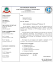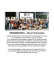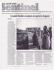# Cellular Capacity Maximization via Robust

## Transcription

Cellular Capacity Maximization via Robust
```Cellular Capacity Maximization via Robust
Venkatesh Chebolu and Siddharth Deshmukh, Member, IEEE
Abstract—In this paper we propose an optimized multi-cell
downlink beamforming solution in which our objective is to
maximize capacity of a cellular network. We first formulate
an optimization problem, maximizing the received signal power
of every active user in a cell, subjected to limiting the overall
interference observed by other users below a specified level. In
addition, we also put constraint on maximum transmit power of
the serving base station. Next, in order to compute robust beamforming vector we accommodate channel estimation error in our
formulation. We consider channel imperfection as error between
true and estimated channel coefficients and we assume error is
bounded with in an ellipsoidal set. The resulting formulation is a
non-convex optimization problem and to get a tractable solution
we exploit linear matrix inequality based S-procedure. The final
reformulation is solved by using semi definite relaxation. The
efficacy of proposed solution in improving cellular capacity and
efficient power transmission is shown by simulations.
Keywords- Downlink beamforming, Semi definite programing,
Cellular capacity maximization.
I.
I NTRODUCTION
Beamforming is a powerful and efficient technique to
transmit and receive the desired signal in presence of cochannel interference by exploiting spatial diversity . In
cellular networks downlink beam forming is used to transmit
the data from the base station (BS) to desired user by deploying
multiple antennas at the serving BS. In addition to co-channel
interference mitigation, efficient beamformer design can improve downlink system capacity and minimize total transmit
power . One way to achieve efficient beam- former design
is coordination between neighbouring BSs . This technique
is also called co-ordinated multipoint transmission (CoMP).
CoMP has been widely recognized as a spectrally efficient
technique which gives high data rates to user terminals in
a cellular network. In a perfect CoMP central unit plays an
important role for the purpose of coordination between the
BSs. The central unit does all signal processing tasks with
BSs sharing their data and global CSI . However such a
centralized processing requires an additional resources of ideal
black haul which is prohibited in practical system. Distributed
or decentralized CoMP systems have become a recent research
Venkatesh Chebolu is with the Department of Electronics and Communication Engineering, National Institute of Technology Rourkela, India. (Email
ID: [email protected])
Siddharth Deshmukh is with the Department of Electronics and Communication Engineering, National Institute of Technology Rourkela, India. (Email
ID: [email protected])
interest as we have practical limitation in centralized CoMP
. Acquisition of CSI at the transmitter i.e. BS (CSIT) in
downlink beamforming in COMP system is very essential
for effective downlink beamforming towards the desired user
terminal. But even with sophisticated training, estimated CSI
cannot be perfect in the practical systems due to several
reasons like estimation error, delay and quantization errors.
Hence in addition to design of decentralized CoMP system it
is important to find the robust design methodologies against
imperfect channel knowledge for accomplishing the effective
gains of CoMP in practical scenarios. In robust formulation
of cellular beamforming problem the true CSIT is considered
to be confined within an uncertainty region and beamforming
vectors are designed such that they remain feasible despite
the imperfections in estimated channel . Such problems
typically lead to optimization problems with infinite number
of constraints and reformulating them to tractable equivalent
Recently many robust methodologies against channel imperfections have been developed for cellular networks. For
example, authors in  have proposed multicellular downlink
beam former design with the objective of minimizing the sum
power used by each BS to transmit data to local users. In
addition CSI imperfection is considered and signal to interference plus noise ratio (SINR) is maintained above the desired
level. Solution for the above problem has been obtained with
the help of S-procedure and semi definite relaxation (SDR). In
 authors studied multi-cell wireless networks and especially
focussed on joint robust transmission optimization over the
cells. They aimed at maximizing the minimum worst case
rate of the network with imperfect CSI. Optimized solution
has been achieved by converting the problem in to convex
problem and solving it by employing convex optimization
problem solvers. In  authors minimize the total transmit
power at BSs by taking equivocation rates and individual SINR
as constraints. In  authors minimize the total downlink
power at BSs by restricting the SINR outage probability below
a threshold.
In this paper, we considers a decentralized CoMP approach
in which each BS with in a cell only sends data to its local
user. This technique helps in reducing the signalling overhead
compared with centralized CoMP. Here we formulate a robust
distributed optimization problem that maximizes the received
signal power of each user present in a particular cell subject
to total transmitted power at the base station remains below
the required level and overall interference on the other cell
users due to the transmission of BS in given cell should be
maintained below a desired level in the presence of imperfect
CSI. We considers the imperfections in CSI between true and
estimated channel coefficients is confined within a spherical
uncertainty set. The worst case solution of the above proposed
problem can be obtained by reformulating non convex problem
as semi definite relaxation and constraints as linear matrix
inequalities. The rest of the paper is structured as follows.
In section-II we provide the system model and problem formulation where non convex formulation is presented. SectionIII develops the robust downlink beamforming formulation in
the presence of imperfect CSI and later we reformulate the
non- convex problem in to convex problem by employing semi
definite relaxation. Simulation results are shown in the section
IV. Finally conclusion is presented in section V.
Notations: In this paper, Rn , C n and H n are used to
denote the sets of n dimensional real, complex vectors and
complex Hermitian matrices, respectively. W and w (or W)
are employed to describe a matrix and vector. The notation
(.)H , (.)T and T r(A) indicate the conjugate transpose ,the
transpose and trace of matrix A. E(.) and k.k provides the
expectation operation and Euclidean norm respectively.
II.
SYSTEM MODEL AND PROBLEM
FORMULATION
We consider a cellular network with N cells. We assume
that each cell in cellular network consists of one base station
and each base station in the network is equipped with M
no of antennas. Further we consider L active single antenna
users in each cell of the network. In the cellular network each
BS directs the beam to its corresponding users. Objective of
the directed beams is to increase the received signal power
of desired user and to restrict the inter-cell interference to
the other users below a particular value. In addition, it also
maintain the total signal power transmitted by the BS below
a particular threshold. Let, Sl = {1, 2, ......U } is the set of
locally active users in particular cell q and So = {1, 2, ......R}
the set of user in adjacent cells which are subjected to intercell interference due to the transmission by the q-th BS with
in the network. Here we define, hi ∈ C M ×1 as the channel
vector which consists of channel coefficients between the BS
in cell q and active users i ∈ Sl , gt ∈ C M ×1 as channel
vector which consists of channel coefficients between the q-th
BS and users t ∈ So . The received signal at user i is given by,
yi = hH
i wi si +
U
X
hH
i wj sj + vi + ni ,
(1)
j=1,j6=i
where, wi ∈ C M ×1 is the beamforming vector corresponding
to the i -th user and si is the complex scalar which denotes
the data symbol for user i . The resultant inter-cell interference
observed at user i because of the transmission by BSs in the
cells other than q is denoted by vi . ni denotes the circularly
symmetric complex Gaussian random variable at user i which
has zero mean and variance σ 2 , ni ∼ CN (0, σ 2 ). We also
assume that the average energy of the transmitting symbol si
2
is normalized to unity i.e Esi (|si | ) = 1. The expression for
the signal to interference plus noise ratio observed by user i
can be written as
H 2
h w i SIN Ri = P i 2
,
(2)
H + ξi + σ 2
j6=i hi wi
where, ξi in above expression is total inter-cell interference
experienced by user i due to the BSs in other cells i.e
2
ξi = E[|vi | ]. Next, to maximize the capacity for the desired
user, we formulate an optimization problem maximizing the
SINR while satisfying the transmit power constraint. Since
maximizing SINR is non convex we maximize the received
signal power while restricting the undesired interference power
to other users. Thus, if every BS restricts interference power
to some threshold, the optimization problem to calculate the
optimum downlink beamforming vectors at any given BS q ,
can be expressed as
2
max hH
i wi
wi
P
P
H
H
(3)
s.t
t∈So
i∈Sl µt wi gt gt wi ≤ K
P
H
i∈Sl ηi wi wi ≤ pq ,
where, K is the maximum allowed interference power at active
users t ∈ So due to the transmission of BS q. pq is the
maximum allowed transmitted signal power by the BS q.
The weighting factor needed for the active user i ∈ Sl is
represented by ηi and µt required for the adjacent outer-cell
users t ∈ So by the BS q. These coefficients are used by
the scheduler for setting up the priority levels which depends
on the quality (cost) of requested services by different users
or to proportionally maintain fairness among the users. The
objective function in (3) denotes the received signal power at
user i , i.e. i ∈ Sl and left side term of inequality in constraint
one in (3) is the overall interference power on the other cell
users except users in Sl due to the transmission of BS q.
Finally left side term of inequality in constraint 2 in (3) is the
total signal power transmitted by the BS q. In next section,
we modify our formulation for robust solution by considering
imperfect channel state information .
FORMULATION IN THE PRESENCE OF CSI
ˆ i ∈ C M ×1 be the
Let us assume, gˆt ∈ C M ×1 and h
estimated CSIs at the BSs. Then the true CSI can be expressed
as
∀t ∈ So ,
∀i ∈ Sl ,
gt = gˆt + et ,
ˆ i + ei ,
hi = h
(4)
where, et ∈ C M ×1 and ei ∈ C M ×1 denotes the CSI error
vectors. We considered that the vectors et and et are bound
with in a ellipsoidal sets defined as
eH
t Qt et ≤ 1,
∀t ∈ So ,
(5)
eH
i Qi ei ≤ 1,
∀i ∈ Sl ,
(6)
where, Qt ∈ C M ×M and Qi ∈ C M ×M are a positive semi
definite matrix which characterizes the shape and size of the
ellipsoid. Substituting for gt and hi from (4) in (3), we will
get
2
ˆH
max
min
hi + eH
)wi (
i
H
wi
s.t
ei Qi ei ≤1
min
eH
i Qi ei ≤1
P
t∈So
(ˆ
gt +
P
H
i∈Sl ηi wi wi
P
i∈Sl
et )(ˆ
gtH
µt wiH
+
eH
t )wi
≤ pq , ∀i ∈ Sl
(7)
≤K
rewritten as
By introducing slack variable z, we can write (7) as
min − z
s.t
Υi ≥ 0,
∀i ∈ Sl ,
Υt ≥ 0,
∀t ∈ So
λi ≥ 0,
∀i
λt ≥ 0,
∀t
Rank(Wi ) = 1, ∀i ∈ Sl ,
P
Pn
H
i∈Sl
j∈1 ηi rj Wi rj − pq ≤ 0. ∀i ∈ Sl
max z
2
ˆH
(hi + eH
i )wi ≥ z
s.t
eH
∀i ∈ Sl ,
i Qi ei ≤ 1,
P
P
H
min
t∈So
i∈Sl µt wi
(8)
eH
i Qi ei ≤1
(ˆ
gt + et )(ˆ
gtH + eH
t )wi ≤
H
et Qt et ≤ 1, ∀t ∈ So ,
P
H
i∈Sl ηi wi wi ≤ pq , ∀i ∈ Sl
K
Here we can observe that the rank constraint in (14) is not
convex. The problem in (14) can be solved by removing the
fifth nonconvex constraint and solving the remaining convex
problem using numerical optimization packages, e.g., CVX
solver, and finally keeping only the rank one solutions for Wi .
The problem in (8) and its constraints can be rewritten as
−z
min
ˆH
(h
i
s.t
−
ˆ
+ eH
i )Wi (hi
H
ei Qi ei ≤ 1,
+ ei ) − z ≥ 0,
∀i ∈ Sl ,
IV.
gtH + eH
t )Wi
t∈So
i∈Sl µt (ˆ
(ˆ
gt + et ) + K ≥ 0,
∀t ∈ So ,
eH
t Qt et ≤ 1,
Rank(Wi ) = 1 ∀i ∈ Sl ,
P
Pn
H
i∈Sl
j∈1 ηi rj Wi rj − pq ≤ 0,
P
P
(9)
where, Wi = wi wiH is a positive semi definite matrix with
unit rank. Here rj is the unit identity vector of order n×1
which has 1 at the j -th position and 0 everywhere with n
as beamforming vector length. Next we discuss, linear matrix
inequality based s-procedure to formulate (9) to SDP form.
Lemma1 (s-procedure ): Let φi (e), for i=0, 1, be defined
as
H
φi (e) = eH Ai e + bH
(10)
i e + e bi + ci ,
M ×M
(14)
M
where, Ai ∈ H
, bi ∈ C and ci ∈ R Suppose, there
exists an eˆ ∈ C M such that φ1 (ˆ
e) < 0. Then the following
two conditions are equivalent:
1) φ0 (e) ≥ 0 and φ1 (e) ≤ 0 are satisfied for all e
2) There exists λ ≥ 0 such that
A 0 b0
A 1 b1
+
λ
≥ 0.
(11)
bH
c0
bH
c1
0
1
SIMULATION RESULTS
A. Simulation set up
In this section, we evaluate performance of cellular network
in terms of improvement in capacity of desired user by using
the solution of the problem in (14). In the first step, we
generate the 7-cell cluster and users, in every cell of the
cluster. Fig.1 shows the example of one user distribution with 7
users. Monte Carlo simulations have been performed over the
distribution of one user and we produce 100 uncertain channel
2
realization per user satisfying ket k ≤ ε2 with different
values. Here we employ channel model used in  which is
given as
gt = 10−(128.1+37.6 log10 (1))/20 .Ψt .φt .(ˆ
gt + et )
(15)
where, the distance between the BS and the user is denoted by
l, Ψt and φt are the shadowing and antenna gain respectively.
gˆt and et indicate the estimated CSI and CSI error corresponding to i th user. Here we choose a spherical uncertainty set with
error radius i.e Qb = ε−2 IM for all b = t, i.
The first four constraints of problem (9) can be written as
ˆ H Wi h
ˆi + h
ˆ H Wi ei + eH Wi h
ˆ i + eH Wi ei − z ≥ 0,
h
i
i
i
i
−
P
eH
i Qi ei
− 1 ≤ 0,
P
[µt gˆtH Wi gˆt + gˆtH Wi et +
t∈So
i∈Sl
(12)
∀i ∈ Sl ,
eH
ˆt + eH
t Wi g
t Wi et ] +
H
et Qt et − 1 ≤ 0, ∀t ∈ So ,
K ≥ 0,
(13)
As per Lemma1, the pairs of inequalities in (12) and (13) hold
if and only if there exist λi ≥ 0 and λt ≥ 0 such that the matrix
inequalities in (16), indicated on the top of the following page
hold. From the second inequalities in (12) and (13) one can
understand that the condition φ1 (ˆ
e) < 0 is trivially satisfied.
Hence the optimization problem in (9) can be equivalently
Fig. 1.
An example of user distributions used in monte-carlo simulations
B. Performance evaluation
In this sub-section, we examine the performance of robust
downlink beamforming for the maximization of capacity of
ˆi
Wi + λi Qi
Wi h
Υi =
∀i ∈ Sl ,
ˆ H Wi
ˆ H Wi h
ˆ i − z − λi 0,
h
h
i
i
P
P
P
P
− P
− Pt∈So i∈Sl µt Wi gˆt
t∈So
i∈Sl µt Wi + λt Qt
P
P
Υt =
0,
− t∈So i∈Sl µt gˆtH Wi
− t∈So i∈Sl µt gˆtH Wi gˆt + K − λt
(16)
∀t ∈ S0 ,
the desired user in the presence of imperfect CSI. Fig.2, Fig.3
and Fig.4 illustrates the plot of target power level at BS versus
total capacity of the desired user.
power must be as minimum as possible. But for particular
minimum upper bound for interference power, the capacity
will be saturated. Hence from the results it can be observed
Fig. 2. Total capacity versus targeted transmit power values in 7-cell scenario
with one user per cell and 4 antenna elements per BS for error radius, ε=0.05
Fig. 4. Total capacity versus targeted transmit power values in 7-cell scenario
with one user per cell and 4 antenna elements per BS for error radius, ε=0.5
In Fig.2 we compare the capacity of desired user with
different upper bounds for interference power (K) induced on
the other user by varying the total power at BS in seven cell
scenario with one user per cell and an error radius ε=0.05.
In Fig.3 and Fig.4, we perform similar analysis which has
that solution to the proposed problem gives high capacity and
power efficiency for a cellular network.
V.
CONCLUSION
This paper Maximizes the capacity of the cellular network
by increasing the received signal power and reducing the intercell interference induced from other cell BSs at every user in
the network while satisfying the transmit power constraint in
the presence of imperfect CSI. We formulated an optimization
problem for improving the capacity of the desired user and
later we modify our original non convex problem in to a
tractable formulation with convex constraints and LMI. We
showed that reformulated problem can be solved easily by
using semi definite relaxation. Simulation results have shown
that minimum upper bound for interference power gives more
capacity for the cellular network and at some minimum upper
bound capacity will be saturated. We also observed that higher
value for error radius demands more robust system.
R EFERENCES
Fig. 3. Total capacity versus targeted transmit power values in 7-cell scenario
with one user per cell and 4 antenna elements per BS for error radius, ε=0.1
been done in Fig.2, but with different error radius ε=0.1 and
ε =0.5 respectively. From Fig.2, Fig.3 and Fig.4, it can be
observed as upper bound for interference power increases total
capacity decreases. Hence the upper bound for the interference

A. B. Gershman, N. D. Sidiropoulos, S. Shahbazpanahi, M. Bengtsson,
and B. Ottersten, “Convex optimization-based beamforming: From
receive to transmit and network designs,” IEEE Signal Process. Mag,
vol. 27, no. 3, pp. 62–75, 2010.
 B. K. Chalise, S. Shahbazpanahi, A. Czylwik, and A. B. Gershman,
“Robust downlink beamforming based on outage probability specifications,” Wireless Communications, IEEE Transactions on, vol. 6, no. 10,
pp. 3498–3503, 2007.

S. Han, Q. Zhang, and C. Yang, “Distributed coordinated multi-point
downlink transmission with over-the-air communication,” in Communications and Networking in China (CHINACOM), 2010 5th International
ICST Conference on. IEEE, 2010, pp. 1–5.
 C. Shen, T.-H. Chang, K.-Y. Wang, Z. Qiu, and C.-Y. Chi, “Distributed
robust multicell coordinated beamforming with imperfect csi: an admm
approach,” Signal Processing, IEEE Transactions on, vol. 60, no. 6, pp.
2988–3003, 2012.
 M. K. Karakayali, G. J. Foschini, and R. A. Valenzuela, “Network coordination for spectrally efficient communications in cellular systems,”
Wireless Communications, IEEE, vol. 13, no. 4, pp. 56–61, 2006.
 M. B. Shenouda and T. N. Davidson, “Convex conic formulations of
robust downlink precoder designs with quality of service constraints,”
Selected Topics in Signal Processing, IEEE Journal of, vol. 1, no. 4,
pp. 714–724, 2007.

M. Tshangini and M. R. Nakhai, “Robust downlink beamforming with
imperfect csi,” in Communications (ICC), 2013 IEEE International
Conference on. IEEE, 2013, pp. 4916–4920.
 A. Tajer, N. Prasad, and X. Wang, “Robust beamforming for multicell downlink transmission,” in Information Theory Proceedings (ISIT),
2010 IEEE International Symposium on. IEEE, 2010, pp. 2063–2067.
 J. Ni, Z. Fei, K.-K. Wong, C. Xing, J. Kuang, and K.-F. Tong, “Robust
coordinated beamforming for secure miso interference channels with
bounded ellipsoidal uncertainties,” Wireless Communications Letters,
IEEE, vol. 2, no. 4, pp. 407–410, 2013.
 Q. Li, A. M.-C. So, and W.-K. Ma, “Distributionally robust chanceconstrained transmit beamforming for multiuser miso downlink,” in
Acoustics, Speech and Signal Processing (ICASSP), 2014 IEEE International Conference on. IEEE, 2014, pp. 3479–3483.
```

### Oguejiofor O.S , Nwalozie G.C , Alagbu E and Okafor A.O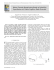### CALL FOR PAPERS Special Session: Software Engineering and its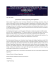### sports splash pots - Gila River Casinos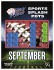### S30A-the cooles power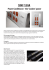### How to do the wiring of XM 300C, XD 312: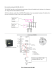### La regla de oro: El coste objetivo del edificio nunca puede ser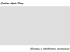### Device Registration Walkthrough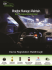### CSI STUDENT BRANCH - Adhiyamaan College of Engineering